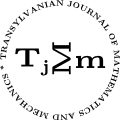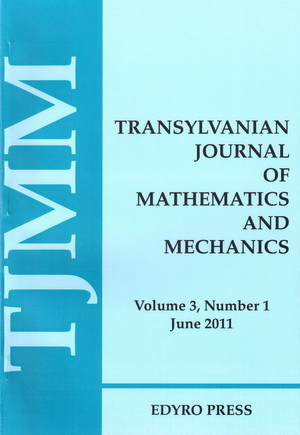ISSN: 2067-239X
ISSN(on-line): 2067-239X

Indexed in:
Mathematical Reviews
Zentralblatt MATH
EBSCO

Front cover# Volume 5 (2013), Number 2

## Vectorial Error Estimates in Approximating Functions of Selfadjoint Operators in Hilbert Spaces by a Perturbed Taylor’s Like Expansion

### Author(s): S.S. DRAGOMIR

Abstract: On utilising the spectral representation of selfadjoint operators in Hilbert spaces, some vectorial error estimates in approximating functions of selfadjoint operators in Hilbert spaces by a perturbed Taylor’s type expansion are given.

## New Fractional Inequalities of Ostrowski Type

### Author(s): ASSIA GUEZANE-LAKOUD and FATIMA AISSAOUI

Abstract: In this work, we establish a new weighted Montgomery identity for Riemann-Liouville fractional integrals. Then using this new fractional Montgomery identity, we obtain some new fractional inequalities of Ostrowski type.

## A Note on Stability Constraints for Asymptotic Stability of the Scalar Equations

### Author(s): ÖZLEM AK GÜMÜŞ and NECATI TASKARA

Abstract: We consider the scalar equation of the form $x\left(n+2k\right)+px\left(n+k\right)+qx\left(n\right)=0$. In the case of $p=1$ or $p=-1$, $q$ constraints have determined for stability of the scalar equation by using the analytical solution. Thus, we have a practical knowledge about the stability of the scalar equation without the need of a computer programming and stability test.

## Heat Capacity of the Relativistic Electron Gas in a Magnetic Field

### Author(s): LAUREAN HOMORODEAN

Abstract: The heat capacity of degenerate and nondegenerate relativistic electron gases in a magnetic field is presented. In both cases, it comprises a term independent of the magnetic induction and a term varying monotonically with the magnetic induction. In the case of the degenerate relativistic electron gas, a third term with an oscillatory dependence on the magnetic induction appears.

## Numerical Solution for Parabolic Equation with Nonlocal Conditions

### Author(s): AHCENE MERAD and ABDELFATAH BOUZIANI

Abstract: In this paper, we study a parabolic equation with purely nonlocal (integral) conditions. We present a numerical approximate solution by Laplace transform method. Some experimental numerical results using the proposed numerical procedure are discussed.

## Some Integral Inequalities for Two Kinds of Convexities Via Fractional Integrals

Abstract: In this paper, we consider the classes of $s$-convex function of first and second kinds respectively. We derive several new Hermite-Hadamard type of inequalities for these classes via fractional integrals.

## Nonuniform Polynomial Trichotomy of Evolution Operators in Banach Spaces

### Author(s): MAGDA LUMINIȚA RĂMNEANȚU

Abstract: In this paper we define the notions of nonuniform polynomial trichotomy and polynomial trichotomy and we emphasize connections between these concepts. The approach is motivated by various examples.

## The Optimality Conditions for the Toll Road Bilevel Programming Problems

### Author(s): CHAOFENG SHI and YINGRUI WANG

Abstract: In a general traffic network, Di Wu, Yafeng Yin and Hai Yang (2011) proved that the level of service, represented by the volume-capacity (v/c) ratio, offered by a profit-maximizing private firm on a private toll road is independent of another competitor’s choice of capacity and toll rate for another private toll road. However, the optimality condition for the toll road bilevel programming is not discussed in Di Wu, Yafeng Yin and Hai Yang (2011). In this paper, the Abadie constraint qualifications and KKT conditions for the toll road bilevel programming is proposed and analyzed, which is the base and prerequisite of the main results in Di Wu, Yafeng Yin and Hai Yang (2011).

$\Delta \left(\right{r}_{n}\Delta \left({x}_{n}+a{x}_{n-\tau }+b{x}_{n+\sigma }\right)\left)\right+{p}_{n}{x}_{n-k}^{\alpha }+{q}_{n}{x}_{n+m}^{\beta }=0$
where $n\in ℕ\left({n}_{0}\right)$. Examples are provided to illustrate the main results.